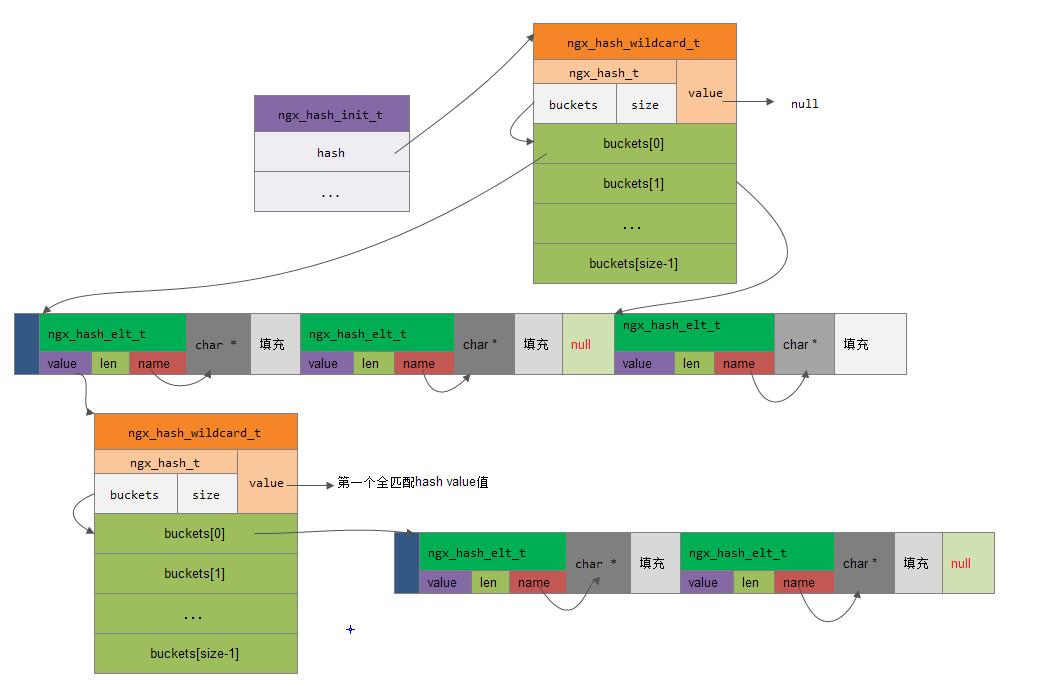• 不带通配符的hash

• 带前向通配符的hash

• 带后向通配符的hash

注意： 不支持同时带有前向通配符与后向通配符的hash


## 1. 函数ngx_hash_wildcard_init(){key = ("com.", 4 ), key_hash = 0, value = "220.181.111.147"}
{key = ("cn.com.baidu.", 13), key_hash = 0, value = "220.181.111.147"}
{key = ("com.baidu.", 10), key_hash = 0, value = "220.181.111.147"}
{key = ("com.google.", 11), key_hash = 0, value = "58.63.236.35"}


{key = ("cn.com.baidu.", 13), key_hash = 0, value = "220.181.111.147"}
{key = ("com.", 4 ), key_hash = 0, value = "220.181.111.147"}
{key = ("com.baidu.", 10), key_hash = 0, value = "220.181.111.147"}
{key = ("com.google.", 11), key_hash = 0, value = "58.63.236.35"}


1) 初始化两个数组

2） 构建Hash表## 2. 函数ngx_hash_key()

key = (ngx_uint_t) key * 31 + data[i];


## 5. 函数ngx_hash_keys_array_init()

• ha->keys: 不带通配符的ngx_hash_key_t数组

• ha->dns_wc_head: 带前向通配符的ngx_hash_key_t数组

• ha->dns_wc_tail: 带后向通配符的ngx_hash_key_t数组

• ha->keys_hash: 这是一个二维数组。该值在调用的过程中用来保存和检测是否有冲突的key值，也就是是否有重复

• ha->dns_wc_head_hash: 这是一个二维数组。该值在调用的过程中用来保存和检测是否有冲突的key值，也就是是否有重复

• ha->dns_wc_tail_hash: 这是一个二维数组。该值在调用的过程中用来保存和检测是否有冲突的key值，也就是是否有重复

1) 判断元素添加到哪一种类的Hash表中

/*
* supported wildcards:
*     "*.example.com", ".example.com", and "www.example.*"
*/


• skip=1: 表示.example.com这种通配类型

• skip=2: 表示*.example.com这种通配类型

• skip=0: 表示www.example.*这种通配类型，此时将last值进行调整last-=2，即减去后面.*两个字符的长度。

2) 插入元素到exact hash中

• 求可以的hash值。 这里如果flags标明的该key不是NGX_HASH_READONLY_KEY不是readonly类型的话，会先将该key转换成小写，然后再求hash值。

• 判断该keyha->keys数组当中是否有重复。 这里可以看到ha->hash_keys哈希表的用处了，就是用于快速判断是否有重复。

• 如果没有重复，则将当前元素<key,value>插入到无通配符的hash表中

3） 检查.example.com类型通配是否在exact hash中

4) 对通配字符串进行相应的格式转换

• skip>0: 表示前置通配字符串。此时*.example.com会被转换成com.example.\0; .example.com会被转换成 com.example\0
• skip=0: 表示后置通配符。此时www.example.*会被转换成www.example\0

5） 在wildcard hash中检查是否有冲突

• *.example.com: 存放的在ha->dns_wc_head_hash中的值为example.com

• .example.com: 存放在ha->dns_wc_head_hash中的值也为example.com

• www.example.*: 存放在ha->dns_wc_tail_hash中的值为www.example

6) 添加转换后的通配字符串到数组中

• *.example.com: 添加到hk中为com.example.

• .example.com: 添加到hk中为com.example

• www.example.*: 添加到hk中为www.example

[参看]